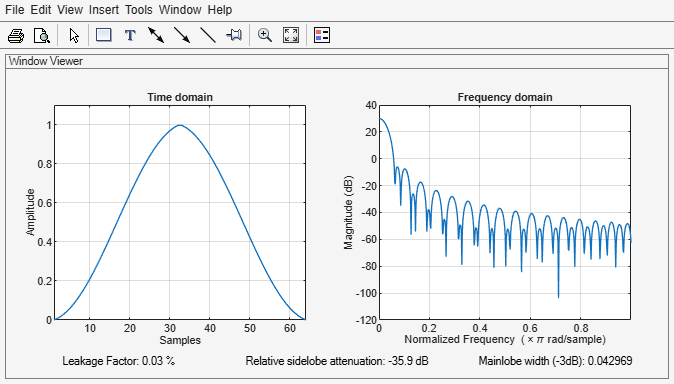barthannwin

Modified Bartlett-Hann window

Syntax

w = barthannwin(L)

Description

w = barthannwin(L) returns an L-point modified Bartlett-Hann window in the column vector w. Like Bartlett, Hann, and Hamming windows, this window has a mainlobe at the origin and asymptotically decaying sidelobes on both sides. It is a linear combination of weighted Bartlett and Hann windows with near sidelobes lower than both Bartlett and Hann and with far sidelobes lower than both Bartlett and Hamming windows. The mainlobe width of the modified Bartlett-Hann window is not increased relative to either Bartlett or Hann window mainlobes.

Note

The Hann window is also called the Hanning window.

Examples

collapse all

Create a 64-point Bartlett-Hann window. Display the result using wvtool.

L = 64;
wvtool(barthannwin(L))Algorithms

The equation for computing the coefficients of a Modified Bartlett-Hanning window is

$w\left(n\right)=0.62-0.48|\left(\frac{n}{N}-0.5\right)|+0.38\mathrm{cos}\left(2\pi \left(\frac{n}{N}-0.5\right)\right)$

where $0\le n\le N$ and the window length is $L=N+1$.

References

 Ha, Y. H., and J. A. Pearce. “A New Window and Comparison to Standard Windows.” IEEE® Transactions on Acoustics, Speech, and Signal Processing. Vol. 37, Number 2, 1999, pp. 298–301.

 Oppenheim, Alan V., Ronald W. Schafer, and John R. Buck. Discrete-Time Signal Processing. Upper Saddle River, NJ: Prentice Hall, 1999, p. 468.